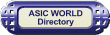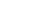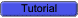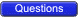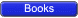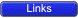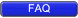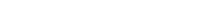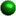Introduction Digital electronics is classified into combinational logic and sequential logic. Combinational logic output depends on the inputs levels, whereas sequential logic output depends on stored levels and also the input levels.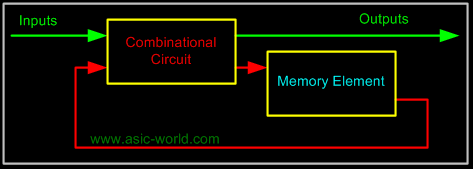The memory elements are devices capable of storing binary info. The binary info stored in the memory elements at any given time defines the state of the sequential circuit. The input and the present state of the memory element determines the output. Memory elements next state is also a function of external inputs and present state. A sequential circuit is specified by a time sequence of inputs, outputs, and internal states.There are two types of sequential circuits. Their classification depends on the timing of their signals:Synchronous sequential circuits Asynchronous sequential circuitsAsynchronous sequential circuit This is a system whose outputs depend upon the order in which its input variables change and can be affected at any instant of time.Gate-type asynchronous systems are basically combinational circuits with feedback paths. Because of the feedback among logic gates, the system may, at times, become unstable. Consequently they are not often used.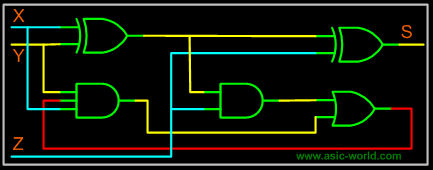Synchronous sequential circuits This type of system uses storage elements called flip-flops that are employed to change their binary value only at discrete instants of time. Synchronous sequential circuits use logic gates and flip-flop storage devices. Sequential circuits have a clock signal as one of their inputs. All state transitions in such circuits occur only when the clock value is either 0 or 1 or happen at the rising or falling edges of the clock depending on the type of memory elements used in the circuit. Synchronization is achieved by a timing device called a clock pulse generator. Clock pulses are distributed throughout the system in such a way that the flip-flops are affected only with the arrival of the synchronization pulse. Synchronous sequential circuits that use clock pulses in the inputs are called clocked-sequential circuits. They are stable and their timing can easily be broken down into independent discrete steps, each of which is considered separately.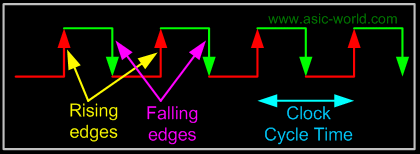A clock signal is a periodic square wave that indefinitely switches from 0 to 1 and from 1 to 0 at fixed intervals. Clock cycle time or clock period: the time interval between two consecutive rising or falling edges of the clock.Clock Frequency = 1 / clock cycle time (measured in cycles per second or Hz)Example:Clock cycle time = 10ns clock frequency = 100Mhz